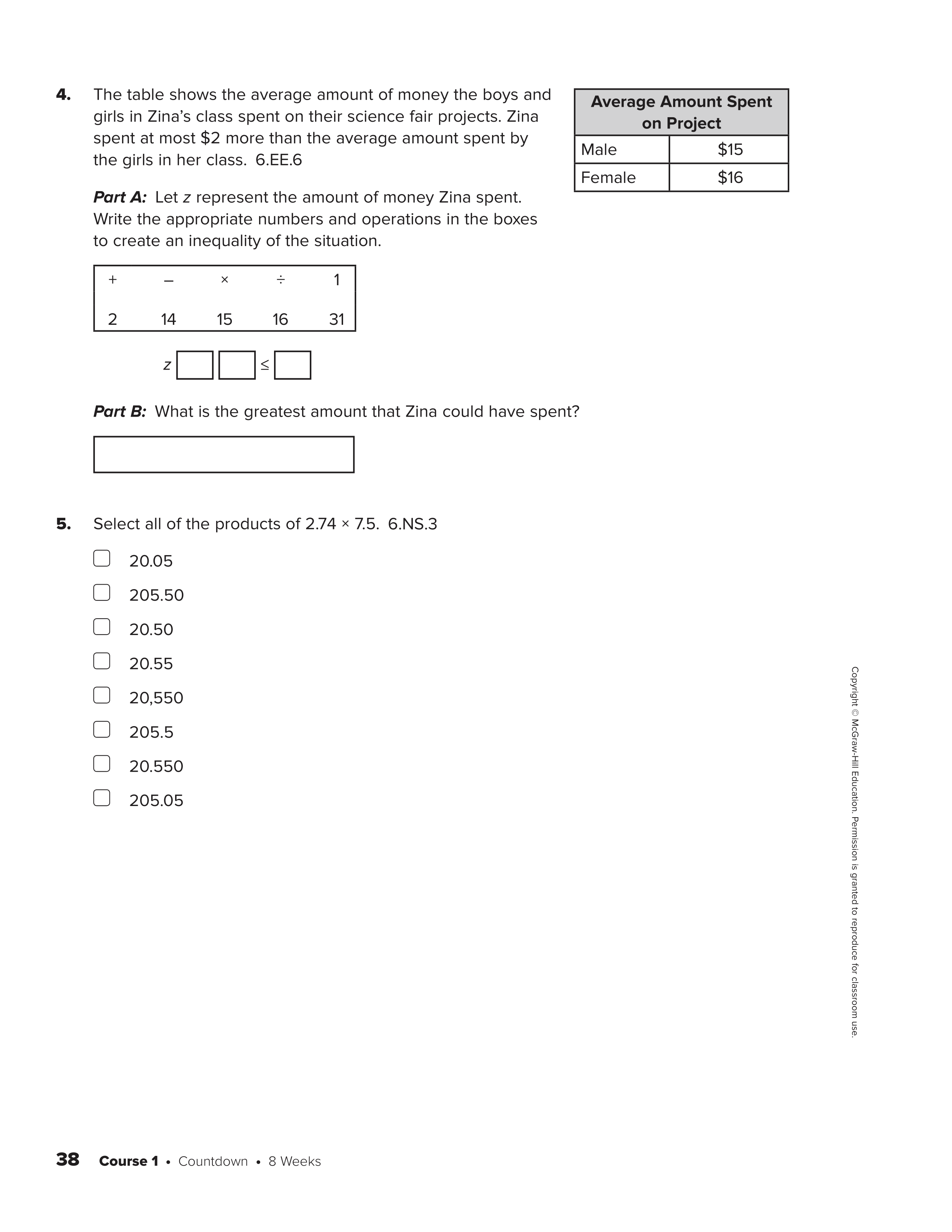SBAC Review - Day 13
starstarstarstarstarstarstarstarstarstar
by Men Sam
| 15 Questions

# 1. Use mental math, calculator, and scratch paper when necessary.

1
1
Statistical questions will give you many numbers, not just one number. Once you have the data set, you can find the mean, median, mode, etc...

Is this a Statistical Question?: How old were you in 3rd grade?

2
1
Statistical questions will give you many numbers, not just one number. Once you have the data set, you can find the mean, median, mode, etc...

Is this a Statistical Question?: How many times have you been to Disneyland?

3
1
Statistical questions will give you many numbers, not just one number. Once you have the data set, you can find the mean, median, mode, etc...

Is this a Statistical Question?: How many times have students been to Disneyland in Mrs. Zuniga's class?

4
1
Find the area of a triangle with these dimensions:

A = ?
b = 4 m
h = 6 m

Hint: Use A = 1/2bh

5
1
Order of Operations:
1. ( )
2. Exponents
3. Multiply or Divide, left one first
4. Add or Subtract, left one first

6
1
Order of Operations:
1. ( )
2. Exponents
3. Multiply or Divide, left one first
4. Add or Subtract, left one first

7
1
Order of Operations:
1. ( )
2. Exponents
3. Multiply or Divide, left one first
4. Add or Subtract, left one first

8
1
1. In a recent survey, 15 skydivers said that they have never had a skydiving accident. This was 60% of the total number of skydivers that were surveyed. What was the total number of skydivers surveyed?

Hint: Set it up as a proportion like this... 15/? = 60/100 (solve by cross multiplying or scaling)

9
1
2. Last year, 39,506 students applied to Harvard University. 5.2% of those students were accepted. How many students did Harvard University accept last year?

Hint: Setup a proportion... ?/39,506 = 5.2/100 (scale or cross multiply)

10
1
3. The University of Minnesota women’s hockey team won 63% of their games this season. Since they won 24 games, how many total games did they play this season?

Hint: Setup a proportion...

# 2. Try your best and submit.11
12
13
11
1
Hint: Statistical questions will give you many numbers, not just one number.

Sample Answer: Y, Y, Y, N
12
1
Hint 1: a square will have the same length on all sides.

Hint 2: 48 = 0.75wh

Sample Answer: w = 6 ft ; l = 6 ft
13
1
Area of a Triangle: A = 1/2bh

[yes or no] ; A + B = [#] square ft ; C = [#] square ft14
15
14
1